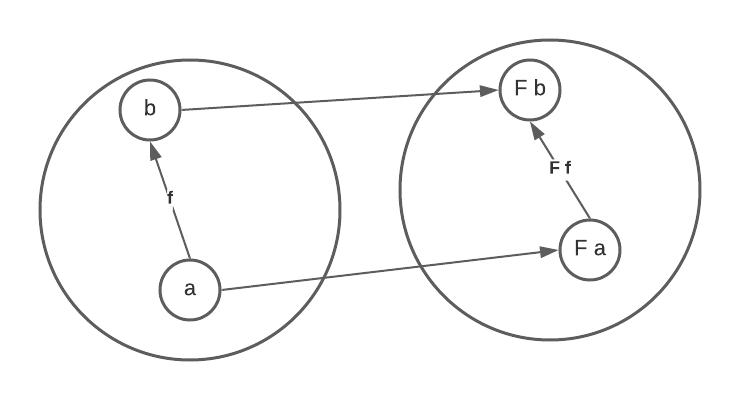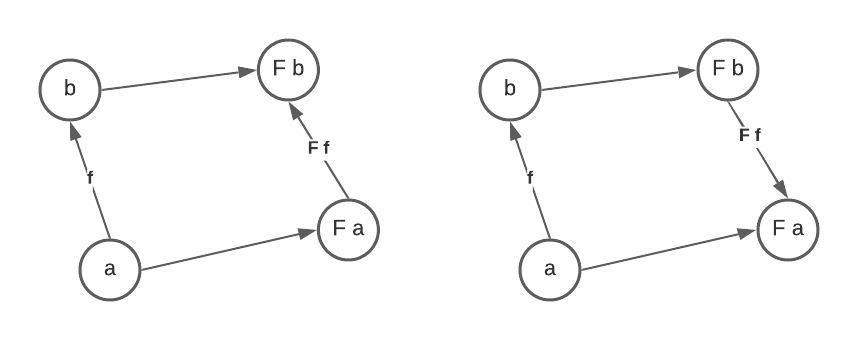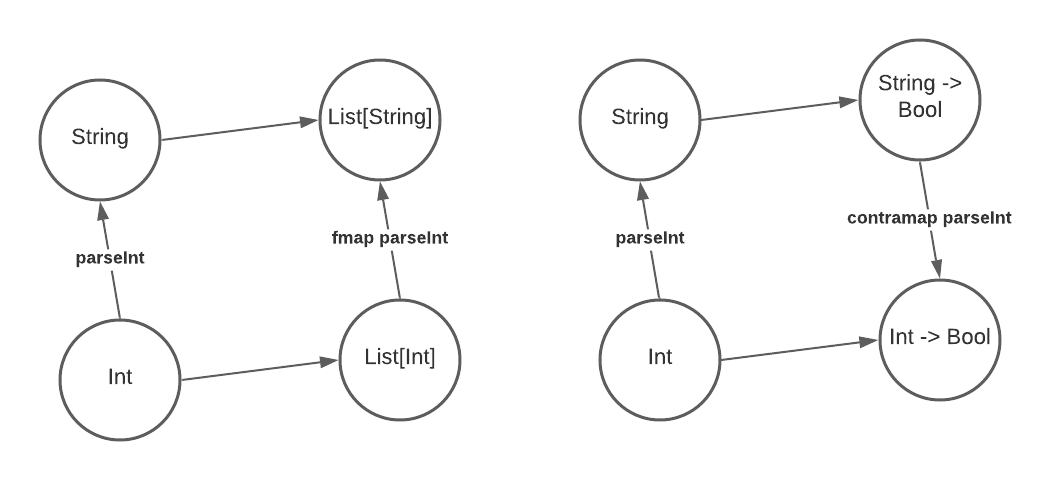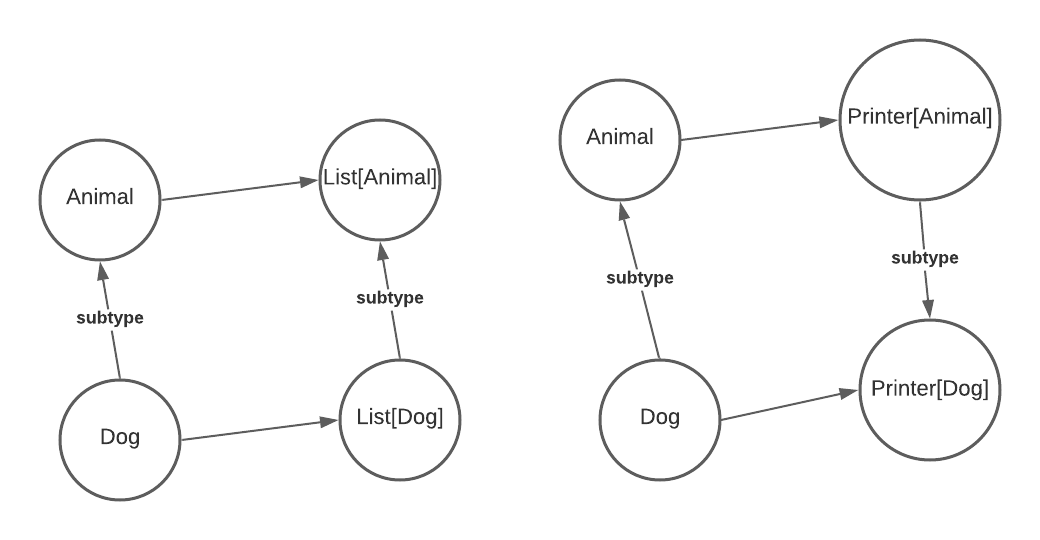## Covariance and Contravariance, functionally

From Covariance and Contravariance we know that generic classes can be made covariant or contravariant. In fact, the same goes for functors. Consider the following type constructor List in Haskell.

data List a = Nil | Cons a (List a)


It’s a covariant functor because we could define a function fmap which takes a function from a to b and returns another function from List a to List b.

fmap :: (a -> b) -> (List a -> List b)


This is the definition of fmap

instance Functor List where
fmap _ Nil = Nil
fmap f (Cons x t) = Cons (f x) (fmap f t)


A contravariant functor is, by contrast, a type constructor f which we could define a contramap taking a function b -> a and returning another one f a -> f b.

contramap :: (b -> a) -> (f a -> f b)


An example of contravariant functors is the so called Writer type, which is essentially a function when the return type is fixed, or, Op r as defined below.

type Op r a = a -> r


The contramap is defined as

instance Contravariant (Op r) where
-- (b -> a) -> Op r a -> Op r b
-- here f is the b -> a and g is the Op r a (or a -> r)
contramap f g = g . f


Notice that f applies before g. Since f is b -> a and g is Op r a (or a -> r) and the result type is Op r b (or b -> r), we should apply f first to convert b to a and then g which converts a to r. In other words, the contramap function of the writer type “inserts” the function b -> a before the function a -> r.

## Covariance and Contravariance, categorically

We know that in category theory, a functor is a mapping between categories that preserves their structures. A functor $$F$$ between two categories is shown below.However, in programming we generally have only one category. Below is how covariant and contravariant functors work in only one category. A covariant functor maps a -> b to F a -> F b, and a contravariant functor maps a -> b to F b -> F a.Note that the word functor is used in both category theory and functional programming. To avoid confusion I refer to functors in functional programming as functional functors. When a single word functor is used, it means functors in category theory.

Below is how covariant and contravariant functors work from the categories perspective. Here the objects are types (like Int and String) and the morphisms are functions between types (there are a lot of morphisms between Int and String, but only parseInt is shown as an example). In this example, both List and Op Bool (a -> Bool) are functors.The List functor maps any type a to type List a and any function f :: a -> b to fmap f :: List a -> List b by applying function f to every element of the list. It preserves the direction of morphisms so it’s covariant. In contrast, the Op Bool functor maps any type a to function type a -> Bool and any function f :: a -> b to contramap f :: (b -> Bool) -> (a -> Bool) by “inserting” itself before the b -> Bool function. It reverses the direction of morphisms so it’s called contravariant.

On the other hand, below is how covariant and contravariant generics work. Here the objects are still types, but the morphisms are subtype relations. In contrast to functional functors, there are only at most one morphism between objects in this category. In this example, both List and Printer are functors, but works on a different category than the example above.The List functor maps any type a to type List[a] and any subtype relation a < b to List[a] < List[b] (here < means “subtype of”). It preserves the direction of morphisms so it’s covariant. In contrast, the Printer functor maps any type a to Printer[a] and any relation a < b to Printer[b] < Printer[a]. It reverses the direction of morphisms so it’s called contravariant.

In conclusion, Both functional functors and generic classes are actually functors, and the words covariant and contravariant in both functors and generics essentially describe the covariance/contravariance of the underlying categorical functors. However, they work on different categories. For functors the morphisms are functions but for generics the morphisms are subtype relations.